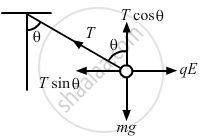Advertisement Remove all ads

# A Pendulum Bob of Mass 80 Mg and Carrying a Charge of 2 × 10−8 C is at Rest in a Uniform, Horizontal Electric Field of 20 Kvm−1. Find the Tension in the Thread. - Physics

Short Note

A pendulum bob of mass 80 mg and carrying a charge of 2 × 10−8 C is at rest in a uniform, horizontal electric field of 20 kVm−1. Find the tension in the thread.

Advertisement Remove all ads

#### Solution

Given:
Magnitude of the charge, q =  2.0 × 10−8 C
Mass of the bob, m = 80 mg = 80 × 10−6 kg
Electric field, E = 20 kVm−1 = 20 × 103 Vm−1
Let the direction of the electric field be from the left to right. Tension in the string is T.
From the figure,Tcosθ = mg    ...(1)
Tsinθ = qE    ...(2)

$\Rightarrow \tan\theta = \frac{qE}{mg}$

$= \frac{20 \times {10}^3 \times 2 \times {10}^{- 8}}{80 \times {10}^{- 6} \times 9 . 8}$

$\Rightarrow \tan\theta = \frac{5}{9 . 8}$

$\Rightarrow \theta = 27^\circ$

From equation (1),

$T = \frac{mg}{cos\theta} = \frac{80 \times {10}^{- 6} \times 9 . 8}{\cos27^\circ}$

$\Rightarrow T = 8 . 8 \times {10}^{- 4}$N

Concept: Force Between Two Point Charges
Is there an error in this question or solution?
Advertisement Remove all ads

#### APPEARS IN

HC Verma Class 11, Class 12 Concepts of Physics Vol. 2
Chapter 7 Electric Field and Potential
Q 46 | Page 122
Advertisement Remove all ads

#### Video TutorialsVIEW ALL 

Advertisement Remove all ads
Share
Notifications

View all notifications

Forgot password?